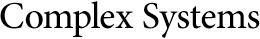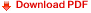## Franklin Squares: A Chapter in the Scientific Studies of Magical SquaresPeter D. Loly
Electronic mail address: loly@cc.umanitoba.ca
Department of Physics and Astronomy,
The University of Manitoba,
Winnipeg, MB Canada R3T 2N2

#### Abstract

Several aspects of magic(al) squares studies fall within the computational universe. Experimental computation has revealed patterns, some of which have lead to analytic insights, theorems, or combinatorial results. Other numerical experiments have provided statistical results for some very difficult problems.

While classical nth order magic squares with the entries 1..n² must have the magic sum for each row, column, and the main diagonals, there are some interesting relatives for which these restrictions are increased or relaxed. These include: serial squares of all orders with sequential filling of rows which are always pandiagonal (i.e., having all parallel diagonals to the main ones on tiling with the same magic sum, also called broken diagonals); pandiagonal logic squares of orders 2n derived from Karnaugh maps; Franklin squares of orders 8n which are not required to have any diagonal properties, but have equal half row and column sums and 2-by-2 quartets; as well as sets of parallel magical bent diagonals.

Our early explorations of magic squares, considered as square matrices, used Mathematica® to study their eigenproperties. We have also studied the moment of inertia and multipole moments of magic squares and cubes (treating the numerical entries as masses or charges), finding some elegant theorems. We have also shown how to easily compound smaller squares into very high order squares. There are patents proposing the use of magical squares for cryptography. Other possible applications include dither matrices for image processing and providing tests for developing constraint satisfaction problem (CSP) solvers for difficult problems.